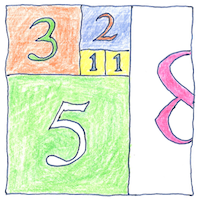# 1202 Number system

## Number system

In Liber Abaci, Leonardo Pisano Bigollo, known today as Fibonacci, the son of Bonacci, popularized the use of the nine numerals, the zero sign, and the concept of place value. In this book (the “Book of Calculation”), Fibonacci showed how to solve business problems— conversions of currency and measurements, and calculations of profit and interest. Fibonacci also showed how to use the number system for purely mathematical concepts— perfect numbers, primes, and series including the eponymous Fibonacci series. Finally Fibonacci described numeric and geometric approximations and irrational numbers such as the square root of two.

## Exchange

The young Leonardo Pisano Bigollo was introduced to the Hindu-Arabic number system in Béjaïa, North Africa, where his father directed a customs house. Businessmen in this case, exchanging both goods and ideas, had been more open than the academics. Subsequently, on business, Leonardo traveled to Egypt, Syria, Greece, Sicily, and Provence and learned from the leading Arab mathematicians.

## Fibonacci sequence

One and one is two; one and two is three; two and three is five and so forth; add the last two numbers to get the next to construct the Fibonacci sequence, describing how breeding rabbits increase and how leaves are arranged on a stem. Plus, the ratio of any two successive Fibonacci numbers approximates the golden number, phi, applicable to art, architecture, theories of beauty, or stock market analysis. Given a simple relation, a sequence of simple additions, a pattern emerges.

Fibonacci contributed to Europe’s adoption of arithmetical methods using the modern numeral system, as opposed to using counting boards (like the abacus) with Roman numerals.